## The image of a parabolic lens is projected onto a graph. The image crosses the x-axis at –2 and 3. The point (–1, 2) is also on the parabola

Question

The image of a parabolic lens is projected onto a graph. The image crosses the x-axis at –2 and 3. The point (–1, 2) is also on the parabola. Which equation can be used to model the image of the lens?

y = (x – 2)(x + 3)
y = (x – 2)(x + 3)
y = (x + 2)(x – 3)
y = (x + 2)(x – 3)

in progress 0
1 week 2021-09-05T08:55:18+00:00 1 Answers 0 views 0

1. Given:

The image of a lens crosses the x-axis at –2 and 3.

The point (–1, 2) is also on the parabola.

To find:

The equation that can be used to model the image of the lens.

Solution:

If the graph of polynomial intersect the x-axis at c, then (x-c) is a factor of the polynomial.

It is given that the image of a lens crosses the x-axis at –2 and 3. It means (x+2) and (x-3) are factors of the function.

So, the equation of the parabola is: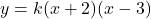…(i)

Where, k is a constant.

It is given that the point (–1, 2) is also on the parabola. It means the equation of the parabola must be satisfy by the point (-1,2).

Putting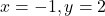in (i), we get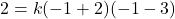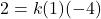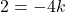Divide both sides by -4.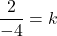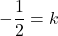Putting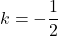in (i), we get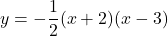Therefore, the required equation of the parabola is.

Note: All options are incorrect.